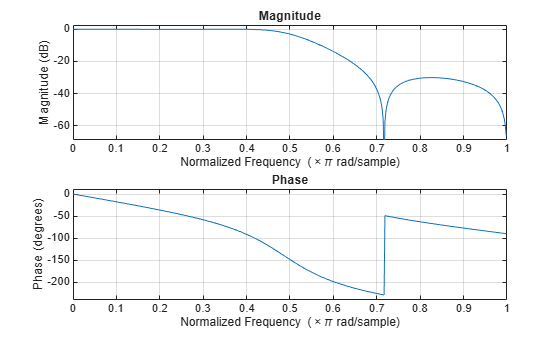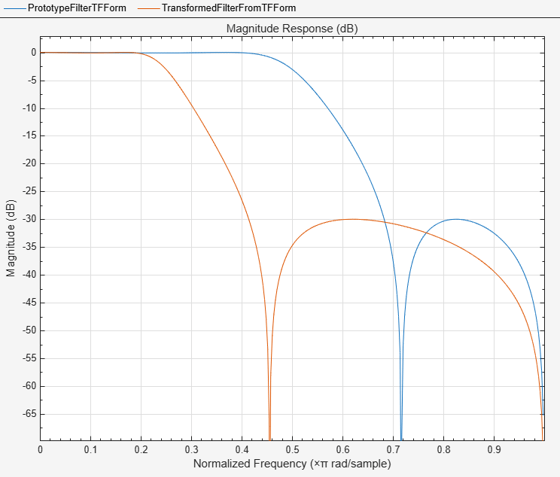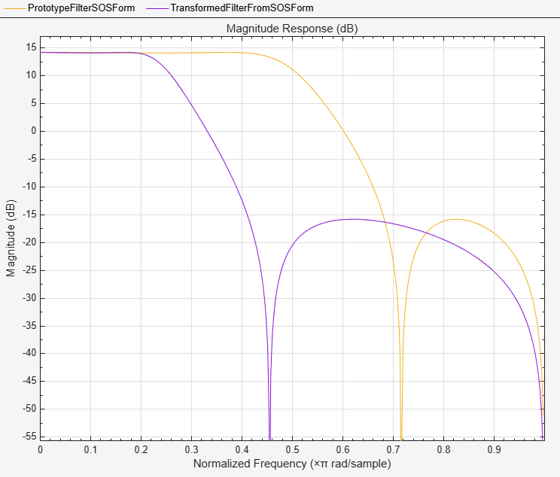# iirftransf

IIR frequency transformation of digital filter

## Syntax

``[num,den] = iirftransf(b,a,allpassNum,allpassDen)``

## Description

example

````[num,den] = iirftransf(b,a,allpassNum,allpassDen)` returns the numerator and the denominator coefficients of the transformed filter. The `iirftransf` function transforms a prototype filter, specified by the numerator `b` and denominator `a`, by using an allpass mapping filter, specified by the numerator `allpassNum` and the denominator `allpassDen`. If you do not specify an allpass mapping filter, then the function returns an original filter.```

## Examples

collapse all

Using the `iirftransf` function, extend the passband of a lowpass IIR filter by using an allpass mapping filter.

Input Lowpass IIR Filter

Design a prototype real IIR lowpass elliptic filter with a gain of about –3 dB at 0.5π rad/sample.

```[b,a] = ellip(3,0.1,30,0.409); fvtool(b,a)```Transform Filter Using `iirftransf`

Extend the passband of the real prototype filter by specifying the allpass mapping filter as a vector of numerator and denominator coefficients, `alpnum` and `alpden` respectively. Use the `allpasslp2lp` function to generate the allpass mapping filter coefficients.

Specify the prototype filter as a vector of numerator and denominator coefficients, `b` and `a` respectively.

```[b,a] = ellip(3,0.1,30,0.409); [alpnum,alpden] = allpasslp2lp(0.5,0.25); [num,den] = iirftransf(b,a,alpnum,alpden);```

Compare the magnitude response of the filters using FVTool.

```fvt = fvtool(b,a,num,den); legend(fvt,"Prototype Filter (TF Form)",... "Transformed Filter")```You can also specify the input lowpass IIR filter as a matrix of coefficients. Pass the second-order section matrices as inputs. The numerator and the denominator coefficients of the transformed filter are given by `num2` and `den2`, respectively.

```ss = tf2sos(b,a); [num2,den2] = iirftransf(ss(:,1:3),ss(:,4:6),... alpnum,alpden);```

Compare the magnitude response of the filters using FVTool.

```hvft = fvtool(ss,[num2 den2]); legend(hvft,"Prototype Filter (SOS Form)",... "Transformed Filter")```## Input Arguments

collapse all

Numerator coefficients of the prototype lowpass IIR filter, specified as either:

• Row vector –– Specifies the values of [b0, b1, …, bn], given this transfer function form:

`$H\left(z\right)=\frac{B\left(z\right)}{A\left(z\right)}=\frac{{b}_{0}+{b}_{1}{z}^{-1}+\cdots +{b}_{n}{z}^{-n}}{{a}_{0}+{a}_{1}{z}^{-1}+\cdots +{a}_{n}{z}^{-n}},$`

where n is the order of the filter.

• Matrix –– Specifies the numerator coefficients in the form of an P-by-(Q+1) matrix, where P is the number of filter sections and Q is the order of each filter section. If Q = 2, the filter is a second-order section filter. For higher-order sections, make Q > 2.

`$b=\left[\begin{array}{ccccc}{b}_{01}& {b}_{11}& {b}_{21}& ...& {b}_{Q1}\\ {b}_{02}& {b}_{12}& {b}_{22}& ...& {b}_{Q2}\\ ⋮& ⋮& ⋮& \ddots & ⋮\\ {b}_{0P}& {b}_{1P}& {b}_{2P}& \cdots & {b}_{QP}\end{array}\right]$`

In the transfer function form, the numerator coefficient matrix bik of the IIR filter can be represented using the following equation:

`$H\left(z\right)=\prod _{k=1}^{P}{H}_{k}\left(z\right)=\prod _{k=1}^{P}\frac{{b}_{0k}+{b}_{1k}{z}^{-1}+{b}_{2k}{z}^{-2}+\cdots +{b}_{Qk}{z}^{-Q}}{{a}_{0k}+{a}_{1k}{z}^{-1}+{a}_{2k}{z}^{-2}+\cdots +{a}_{Qk}{z}^{-Q}},$`

where,

• a –– Denominator coefficients matrix. For more information on how to specify this matrix, see `a`.

• k –– Row index.

• i –– Column index.

When specified in the matrix form, b and a matrices must have the same number of rows (filter sections) Q.

Data Types: `single` | `double`
Complex Number Support: Yes

Denominator coefficients for a prototype lowpass IIR filter, specified as one of these options:

• Row vector –– Specifies the values of [a0, a1, …, an], given this transfer function form:

`$H\left(z\right)=\frac{B\left(z\right)}{A\left(z\right)}=\frac{{b}_{0}+{b}_{1}{z}^{-1}+\cdots +{b}_{n}{z}^{-n}}{{a}_{0}+{a}_{1}{z}^{-1}+\cdots +{a}_{n}{z}^{-n}},$`

where n is the order of the filter.

• Matrix –– Specifies the denominator coefficients in the form of an P-by-(Q+1) matrix, where P is the number of filter sections and Q is the order of each filter section. If Q = 2, the filter is a second-order section filter. For higher-order sections, make Q > 2.

`$a=\left[\begin{array}{ccccc}{a}_{01}& {a}_{11}& {a}_{21}& \cdots & {a}_{Q1}\\ {a}_{02}& {a}_{12}& {a}_{22}& \cdots & {a}_{Q2}\\ ⋮& ⋮& ⋮& \ddots & ⋮\\ {a}_{0P}& {a}_{1P}& {a}_{2P}& \cdots & {a}_{QP}\end{array}\right]$`

In the transfer function form, the denominator coefficient matrix aik of the IIR filter can be represented using the following equation:

`$H\left(z\right)=\prod _{k=1}^{P}{H}_{k}\left(z\right)=\prod _{k=1}^{P}\frac{{b}_{0k}+{b}_{1k}{z}^{-1}+{b}_{2k}{z}^{-2}+\cdots +{b}_{Qk}{z}^{-Q}}{{a}_{0k}+{a}_{1k}{z}^{-1}+{a}_{2k}{z}^{-2}+\cdots +{a}_{Qk}{z}^{-Q}},$`

where,

• b –– Numerator coefficients matrix. For more information on how to specify this matrix, see `b`.

• k –– Row index.

• i –– Column index.

When specified in the matrix form, a and b matrices must have the same number of rows (filter sections) P.

Data Types: `single` | `double`
Complex Number Support: Yes

Numerator coefficients of the mapping filter, specified as a row vector.

Data Types: `single` | `double`
Complex Number Support: Yes

Denominator coefficients of the mapping filter, specified as a row vector.

Data Types: `single` | `double`
Complex Number Support: Yes

## Output Arguments

collapse all

Numerator coefficients of the transformed filter, returned as one of the following:

• Row vector of length n+1, where n is the order of the input filter. The `num` output is a row vector when the input coefficients `b` and `a` are row vectors.

• P-by-(Q+1) matrix, where P is the number of filter sections and Q is the order of each section of the transformed filter. The `num` output is a matrix when the input coefficients `b` and `a` are matrices.

Data Types: `single` | `double`
Complex Number Support: Yes

Denominator coefficients of the transformed filter, returned as one of the following:

• Row vector of length n+1, where n is the order of the input filter. The `den` output is a row vector when the input coefficients `b` and `a` are row vectors.

• P-by-(Q+1) matrix, where P is the number of filter sections and Q is the order of each section of the transformed filter. The `den` output is a matrix when the input coefficients `b` and `a` are matrices.

Data Types: `single` | `double`

## Version History

Introduced in R2011a